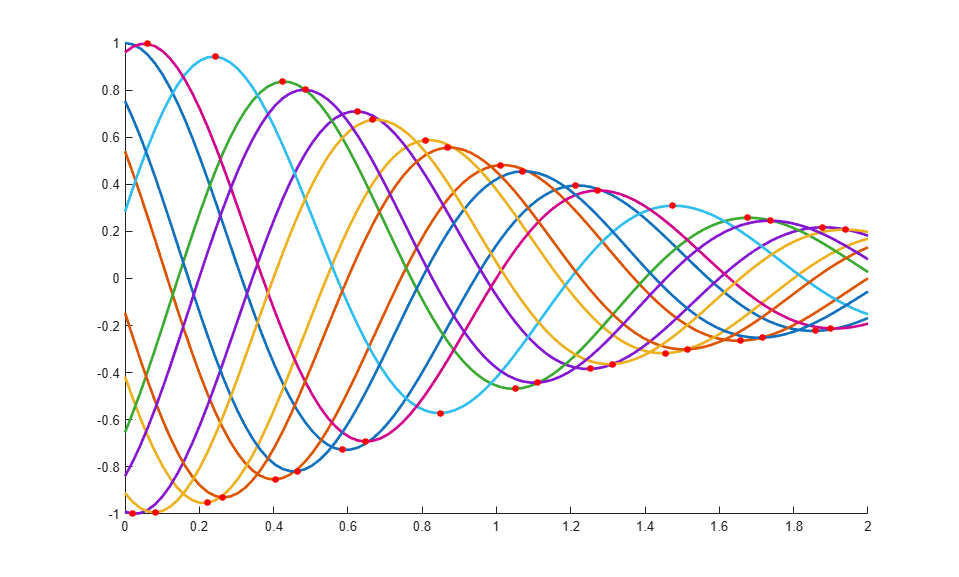# Optimized Chart Class for Displaying Variable Number of Lines

This example shows how to optimize a chart class for displaying a variable number of lines. It reuses existing line objects, which can improve the performance of the chart, especially if the number of lines does not change frequently. For a simpler version of this chart without the optimization, see Chart Class with Variable Number of Lines.

The chart displays as many lines as there are columns in the `YData` matrix, with circular markers at the local extrema. The following code demonstrates how to:

• Define two properties called `PlotLineArray` and `ExtremaLine` that store the objects for the lines and the markers, respectively.

• Implement a `setup` method that initializes the `ExtremaLine` object.

• Implement an `update` method that gets the size of the `PlotLineArray`, and then adds or subtracts objects from that array according to the number of columns in `YData`.

To define the class, copy this code into the editor and save it with the name `OptimLocalExtremaChart.m` in a writable folder.

```classdef OptimLocalExtremaChart < matlab.graphics.chartcontainer.ChartContainer % c = OptimLocalExtremaChart('XData',X,'YData',Y,Name,Value,...) % plots one line with markers at local extrema for every column of matrix Y. % You can also specify the additonal name-value arguments, 'MarkerColor' % and 'MarkerSize'. properties XData (:,1) double = NaN YData (:,:) double = NaN MarkerColor {validatecolor} = [1 0 0] MarkerSize (1,1) double = 5 end properties(Access = private,Transient,NonCopyable) PlotLineArray (:,1) matlab.graphics.chart.primitive.Line ExtremaLine (:,1) matlab.graphics.chart.primitive.Line end methods(Access = protected) function setup(obj) obj.ExtremaLine = matlab.graphics.chart.primitive.Line(... 'Parent', obj.getAxes(), 'Marker', 'o', ... 'MarkerEdgeColor', 'none', 'LineStyle',' none'); end function update(obj) % Get the axes ax = getAxes(obj); % Create extra lines as needed p = obj.PlotLineArray; nPlotLinesNeeded = size(obj.YData, 2); nPlotLinesHave = numel(p); for n = nPlotLinesHave+1:nPlotLinesNeeded p(n) = matlab.graphics.chart.primitive.Line('Parent', ax, ... 'SeriesIndex', n, 'LineWidth', 2); end % Update the lines for n = 1:nPlotLinesNeeded p(n).XData = obj.XData; p(n).YData = obj.YData(:,n); end % Delete unneeded lines delete(p((nPlotLinesNeeded+1):numel(p))) obj.PlotLineArray = p(1:nPlotLinesNeeded); % Replicate x-coordinate vectors to match size of YData newx = repmat(obj.XData(:),1,size(obj.YData,2)); % Find local minima and maxima and plot markers tfmin = islocalmin(obj.YData,1); tfmax = islocalmax(obj.YData,1); obj.ExtremaLine.XData = [newx(tfmin); newx(tfmax)]; obj.ExtremaLine.YData = [obj.YData(tfmin); obj.YData(tfmax)]; obj.ExtremaLine.MarkerFaceColor = obj.MarkerColor; obj.ExtremaLine.MarkerSize = obj.MarkerSize; % Make sure the extrema are on top uistack(obj.ExtremaLine, 'top'); end end end ```

After saving the class file, you can create an instance of the chart. For example:

```x = linspace(0,2)'; y = cos(5*x)./(1+x.^2); c = OptimLocalExtremaChart('XData',x,'YData',y); ```Now, create a `for` loop that adds an additional line to the plot at every iteration. The chart object keeps all the existing lines, and adds one additonal line for each `i`.

```for i=1:10 y = cos(5*x+i)./(1+x.^2); c.YData = [c.YData y]; end ```